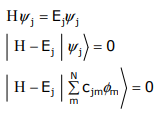# 1.8: N-dimensional cyclic systems

$$\newcommand{\vecs}{\overset { \rightharpoonup} {\mathbf{#1}} }$$ $$\newcommand{\vecd}{\overset{-\!-\!\rightharpoonup}{\vphantom{a}\smash {#1}}}$$$$\newcommand{\id}{\mathrm{id}}$$ $$\newcommand{\Span}{\mathrm{span}}$$ $$\newcommand{\kernel}{\mathrm{null}\,}$$ $$\newcommand{\range}{\mathrm{range}\,}$$ $$\newcommand{\RealPart}{\mathrm{Re}}$$ $$\newcommand{\ImaginaryPart}{\mathrm{Im}}$$ $$\newcommand{\Argument}{\mathrm{Arg}}$$ $$\newcommand{\norm}{\| #1 \|}$$ $$\newcommand{\inner}{\langle #1, #2 \rangle}$$ $$\newcommand{\Span}{\mathrm{span}}$$ $$\newcommand{\id}{\mathrm{id}}$$ $$\newcommand{\Span}{\mathrm{span}}$$ $$\newcommand{\kernel}{\mathrm{null}\,}$$ $$\newcommand{\range}{\mathrm{range}\,}$$ $$\newcommand{\RealPart}{\mathrm{Re}}$$ $$\newcommand{\ImaginaryPart}{\mathrm{Im}}$$ $$\newcommand{\Argument}{\mathrm{Arg}}$$ $$\newcommand{\norm}{\| #1 \|}$$ $$\newcommand{\inner}{\langle #1, #2 \rangle}$$ $$\newcommand{\Span}{\mathrm{span}}$$$$\newcommand{\AA}{\unicode[.8,0]{x212B}}$$

This lecture will provide a derivation of the LCAO eigenfunctions and eigenvalues of N total number of orbitals in a cyclic arrangement. The problem is illustrated below:There are two derivations to this problem.

## Polynomial Derivation

The Hückel determinant is given by,

$D_{N}(x)=\left|\begin{array}{ccccccccc} x & 1 & & & & & & & \\ 1 & x & 1 & & & & & & \\ & 1 & x & \ddots & & & & & \\ & & 1 & \ddots & \ddots & & & & \\ & & & \ddots & \ddots & \ddots & & & \\ & & & & \ddots & \ddots & \ddots & & \\ & & & & & \ddots & \ddots & 1 & \\ & & & & & & \ddots & x & 1 \\ & & & & & & 1 & x \end{array}\right|=0$

where

$x=\frac{\alpha-E}{\beta}$

From a Laplace expansion one finds,

DN(x) = xDn-1(x) - DN-2(x)

WhereWith these parameters defined, the polynomial form of DN(x) for any value of N can be obtained,

D3(x) = xD2(x) – D1(x) = x(x2–1) – x = x(x2–2)

D4(x) = xD3(x) – D2(x) = x2(x2–2) – (x2–1)

$\vdots \nonumber$

and so on

The expansion of DN(x) has as its solution,

$x={-2}\cos \dfrac{2\pi}{N}j (j= 0, 1, 2, 3...N-1) \nonumber$

and substituting for x,

$E = \alpha + 2\beta\cos \dfrac{2\pi}{N}j (j= 0, 1, 2, 3...N-1) \nonumber$

## Standing Wave Derivation

An alternative approach to solving this problem is to express the wavefunction directly in an angular coordinate, θFor a standing wave of λ about the perimeter of a circle of circumference c,

$\psi_j = \sin \dfrac{c}{\lambda} \theta \nonumber$

The solution to the wave function must be single valued ∴ a single solution must be obtained for ψ at every 2nπ or in analytical terms,Thus the amplitude of $$ψ_j$$ at atom m is, (where c/λ = j and θ = (2π/N)m)

$\psi_{j}(m) = \sin{2m\pi}{N}j (j= 0, 1, 2, 3...N-1) \nonumber$

Within the context of the LCAO method, ψj may be rewritten as a linear combination in φm with coefficients cjm. Thus the amplitude of ψj at m is equivalent to the coefficient of φm in the LCAO expansion,

$\psi_{j} = \displaystyle \sum_{k=1}^N C_{jm\phi m}$

Where

$C_{jm} = \sin{2\pi m}{N}j (j= 0, 1, 2, 3...N-1) \nonumber$

The energy of each MO, ψj, may be determined from a solution of Schrödinger’s equation,The energy of the φm orbital is obtained by left–multiplying by φm,but the Hückel condition is imposed; the only terms that are retained are those involving φm, φm+1, and φm-1. Expanding,Evaluating the integrals,Substituting for cjm,

$\alpha \sin \dfrac{2\pi m}{N}j + \beta \left( \sin \dfrac{2\pi (m+1)}{N}j + \sin \dfrac{2\pi (m-1)}{N}j \right) = E_{j} \sin \dfrac{2\pi m}{N}j \nonumber$$\alpha + \dfrac{ \beta \left( \sin \dfrac{2\pi (m+1)}{N}j + \sin \dfrac{2\pi (m-1)}{N}j \right)}{ \sin \dfrac{2\pi m}{N}j} = E_{j} \nonumber$$E_{j} = \alpha + 2\beta \cos k \nonumber$

$E_{j} = \alpha + 2\beta \cos \dfrac{2\pi}{N}j (j= 0, 1, 2, 3...N-1) \nonumber$

Let’s look at the simplest cyclic system, N = 3Continuing with our approach (LCAO) and using Ej to solve for the eigenfunction, we find…Using the general expression for ψj, the eigenfunctions are:

$\psi_{0} = e^{i(0)0} \phi_{1} + e^{i(0) \dfrac{2\pi}{3}} \phi_{2} + e^{i(0) \dfrac{4\pi}{3}} \phi_{3} \nonumber$

$\psi_{1} = e^{i(1)0} \phi_{1} + e^{i(1) \dfrac{2\pi}{3}} \phi_{2} + e^{i(1) \dfrac{4\pi}{3}} \phi_{3} \nonumber$

$\psi_{-1} = e^{i(-1)0} \phi_{1} + e^{i(-1) \dfrac{2\pi}{3}} \phi_{2} + e^{i(-1) \dfrac{4\pi}{3}} \phi_{3} \nonumber$

Obtaining real components of the wavefunctions and normalizing,


\begin{array}{ll}
\psi_{0}=\phi_{1}+\phi_{2}+\phi_{3} \rightarrow & \psi_{0}=\frac{1}{\sqrt{3}}\left(\phi_{1}+\phi_{2}+\phi_{3}\right) \\
\psi_{+1}+\psi_{-1}=2 \phi_{1}-\phi_{2}-\phi_{3} \rightarrow & \psi_{1}=\frac{1}{\sqrt{6}}\left(2 \phi_{1}-\phi_{2}-\phi_{3}\right) \\
\psi_{+1}-\psi_{-1}=\phi_{2}-\phi_{3} \rightarrow & \psi_{2}=\frac{1}{\sqrt{2}}\left(\phi_{2}-\phi_{3}\right)
\end{array}
\]

Summarizing on a MO diagram where α is set equal to 0,This page titled 1.8: N-dimensional cyclic systems is shared under a CC BY-NC-SA 4.0 license and was authored, remixed, and/or curated by Daniel Nocera via source content that was edited to the style and standards of the LibreTexts platform; a detailed edit history is available upon request.